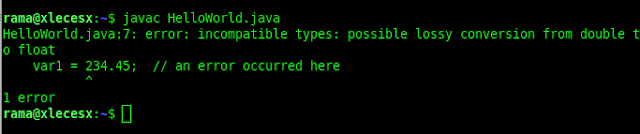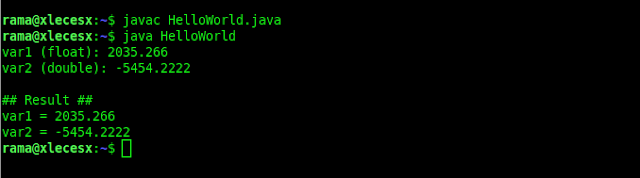# Float and Double Data Type Java Language With ExampleFloat and double data types are fractional number data types. This time we will discuss the difference between float and double in java programming.

### Definition of float and double data types in java language

Float and double data types are used to hold fractional numbers such as 3.14, 44.53, or -0.09876. Just like programming languages in general, we use periods as separators of integers and fractions.

The difference between float and double lies in the range of numbers and the level of accuracy. The following table is the difference between float and double data types in Java:

Although float and double data types can store very large numbers, but this data type has general weaknesses as is the case in every programming language (not only Java). That is the level of accuracy. This relates to the mechanism of storage in computers that use binary numbers.

Example of java and float program code examples.

As the first example java code, I will create 2 variables of type float and double, input numbers, then display them:
``````
class HellWorld {
public static void main(String args[]){

float  var1;
double var2;

var1 = 234.45F;
var2 = 234.45;

System.out.println("var1 = "+var1);
System.out.println("var2 = "+var2);
}
}
``````

Program code results:
``````
var1 = 234.45
var2 = 234.45
``````

At the beginning of the program code, I declare a variable var1 of type float, and variable var2 of type double. Then in lines 7-8, these two variables are filled with numbers 234.45.

Notice how to fill in the variable var1, there is a suffix "F", i.e. 234.45F. This is necessary because by default all fractional numbers in Java are considered double.

Finally these two variables are displayed with the System.out.println() command in lines 10 and 11.

The suffix "F" for this float data type input process must be written, otherwise there will be an error like the following example:
``````
class HelloWorld {
public static void main(String args[]){

float  var1;
double var2;

var1 = 234.45;  // an error occurred here
var2 = 234.45;

System.out.println("var1 = "+var1);
System.out.println("var2 = "+var2);
}
}
``````In this case the Java compiler refuses because there is a conversion process from double to float.

Writing fractional numbers can also use scientific notation, such as 3.12e2 or 4E-3. The character e or E represents the rank of 10, so 3.12e2 = 3.12 x 102 = 312. Whereas 4E-3 = 4 x 10-3 = 0.004. Here is an example:
``````
class HelloWorld {
public static void main(String args[]){

float var1;
double var2;

var1 = 3.12e2F;
var2 = 4E-3;

System.out.println("var1 = "+var1);
System.out.println("var2 = "+var2);
}
}
``````

Program code results:
``````
var1 = 312.0
var2 = 0.004
``````

### How to read (input) Double and Float Data

To input double and float data type processes, you can also use the Scanner class by using the following input commands:
• nextFloat ()
• nextDouble ()
Also to avoid punctuation problems, this input process should also include the Locale class. Here's an example:
``````
import java.util.Scanner;
import java.util.Locale;

class HelloWorld {
public static void main(String args[]){

Scanner input = new Scanner(System.in).useLocale(Locale.US);

float  var1;
double var2;

System.out.print("var1 (float): ");
var1 = input.nextFloat();

System.out.print("var2 (double): ");
var2 = input.nextDouble();

System.out.println();
System.out.println("## Result ##");

System.out.println("var1 = "+var1);
System.out.println("var2 = "+var2);
}
}
``````At the beginning of the program code, there is a code for importing java.util.Scanner and java.util.Locale. This Locale class is needed during the Scanner class initiation process inline 7. It functions so that the input process uses the American system (US), which uses a dot as a fraction separator.

So to be uniform, we just set it with the US system. The reading process itself is carried out in lines 13 and 16 for the float data type and double data type.

### So, should you use float or double data types?

For general use, you should only use double. Aside from being larger in scope, double is also the default fraction data type from Java, so we don't need to add the "F" suffix when we want to fill values into variables.